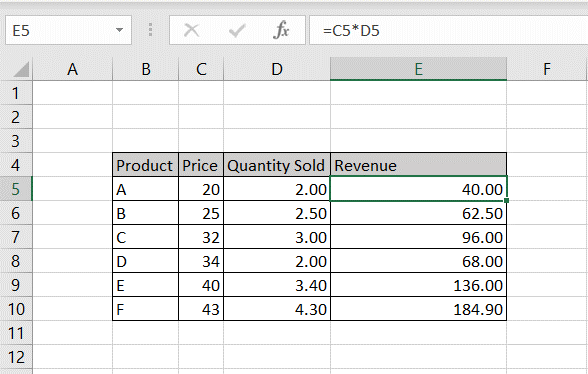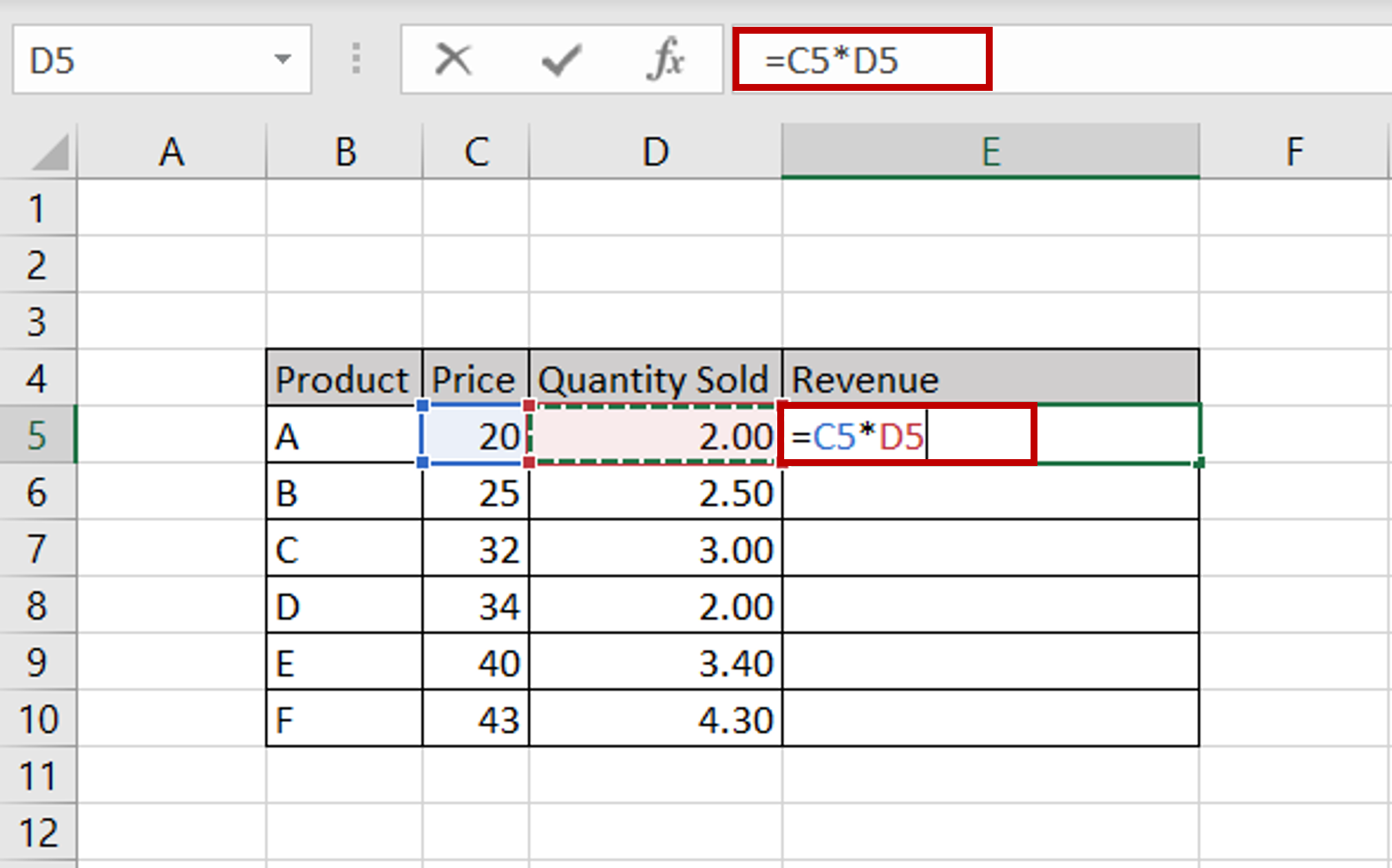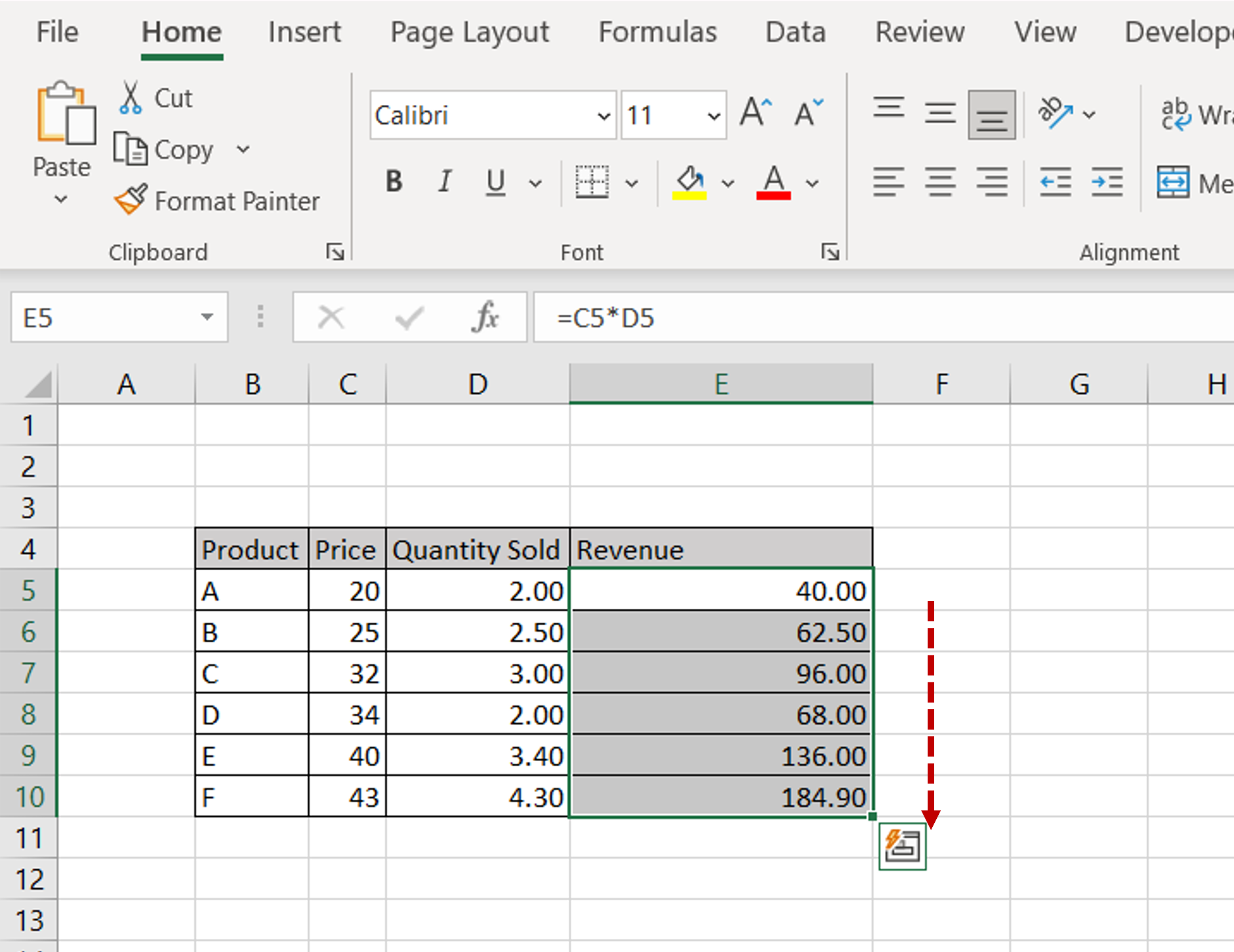# How to multiply two columns in Excel

You can watch a video tutorial here.Excel is widely used for calculations due to the several arithmetic operators and functions that it has. You may need to multiply two columns, for example, if you have the Price and Quantity sold and you need to compute the Revenue.

### Step 1 – Enter the formula– In the first cell of the ‘Revenue’ column, enter the formula:
=<cell reference of the ‘Price’ column> * <cell reference of the ‘Quantity sold’ column>

### Step 2 – Copy the formula– Using the fill handle from the first cell, drag the formula to the remaining cells
OR
a) Select the cell with the formula and press Ctrl+C or choose Copy from the context menu (right-click)
b) Select the rest of the cells in the column and press Ctrl+V or choose Paste from the context menu (right-click)